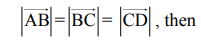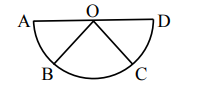# Assertion A : If A, B, C, D are four points on a semi-circular arc with centre at 'O' such that`
Question:

Assertion A : If A, B, C, D are four points on a semi-circular arc with centre at 'O' such that$\overrightarrow{\mathrm{AB}}+\overrightarrow{\mathrm{AC}}+\overrightarrow{\mathrm{AD}}=4 \overrightarrow{\mathrm{AO}}+\overrightarrow{\mathrm{OB}}+\overrightarrow{\mathrm{OC}}$

Reason R : Polygon law of vector addition yields

$\overrightarrow{\mathrm{AB}}+\overrightarrow{\mathrm{BC}}+\overrightarrow{\mathrm{CD}}+\overrightarrow{\mathrm{AD}}=2 \overrightarrow{\mathrm{AO}}$In the light of the above statements, choose the most appropriate answer from the options given below :

1. A is correct but R is not correct.

2. A is not correct but R is correct.

3. Both A and R are correct and R is the correct explanation of A.

4. Both A and R are correct but R is not the correct explanation of A.

Correct Option: , 4

Solution:

Polygon law is applicable in both but the equation given in the reason is not useful in explaining the assertion.